# Vertical Stress in Ground Notes | Study Soil Mechanics - Civil Engineering (CE)

## Civil Engineering (CE): Vertical Stress in Ground Notes | Study Soil Mechanics - Civil Engineering (CE)

The document Vertical Stress in Ground Notes | Study Soil Mechanics - Civil Engineering (CE) is a part of the Civil Engineering (CE) Course Soil Mechanics.
All you need of Civil Engineering (CE) at this link: Civil Engineering (CE)

Stress Distribution in The Soil

At a point within a soil mass, stresses will be developed as a result of the soil lying above the point and by any structural or other loading imposed onto that soil mass.

Stress in the soil may be caused by:

1. Self weight of soil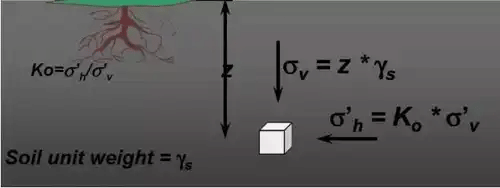If the surface loading area is finite (point, circular, strip, rectangular, square), the vertical stress increment in the subsoil decreases with increase in the depth and the distance from the surface loading area.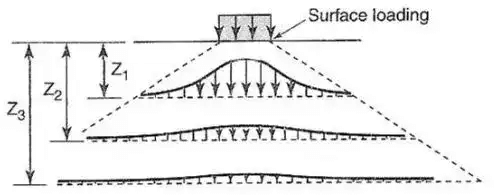Methods have been developed to estimate the vertical stress increment in sub-soil considering the shape of the surface loading area.

Boussinesq's Theory
A point load or a Concentrated load is, strictly speaking, hypothetical in nature, consideration of it serves a useful purpose in arriving at the solutions for more complex loadings in practice.
(i) The soil medium is an elastic, homogeneous, isotropic and semi-infinite medium, which infinitely in all directions from a level surface.
(ii) The medium obeys Hookes law.
(iii) The self-weight of the soil is ignored.
(iv) The soil is initially unstressed
(v) The change in volume of the soil upon application of the loads onto it is neglected.
(vi) The top surface of the medium is free of shear stress and is subjected to only the point load at a specified location.
(vii) Continuity of stress is considered to exist in the medium.
(viii) The stresses are distributed symmetrically with respect to z-axis.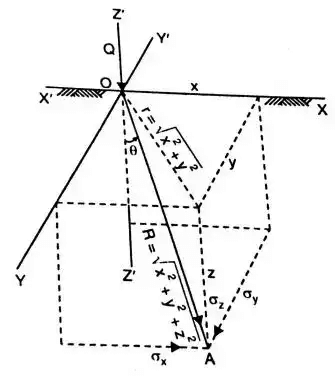The Boussinesq equations are as follows: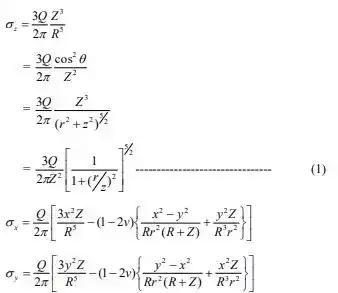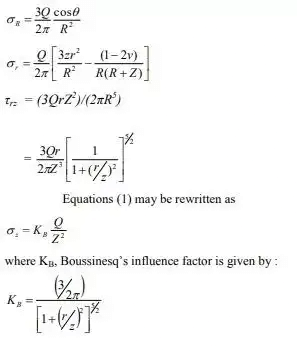This intensity of vertical stress directly below the point load, on its axis of loading (r = 0) is given by:
σz = 0.4775Q / Z2
The vertical stress on a horizontal plane at depth „Z‟ is given by
σ= KB(Q / Z2)
Z being a specified depth.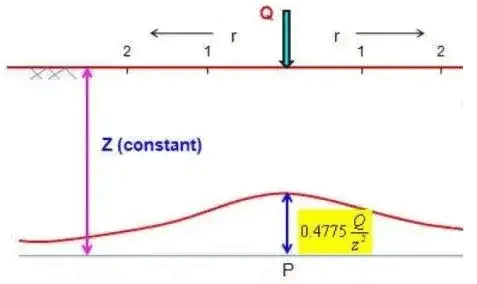3. Boussinesq's Result
σz|max = 0.0888(Q / r2)
σz|max = 0.1332(Q/ 22)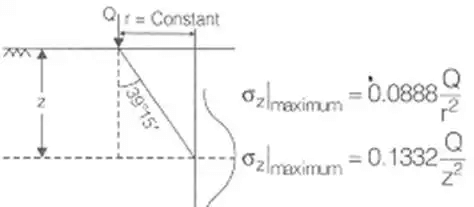Westergaard's Theory

(i)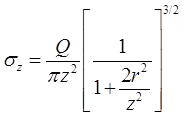(ii) σz= kW.(Q / z2)
(iii) kw|max = 0.3183

1. Westergaard's Results
(i) Vertical Stresss due to Live Loads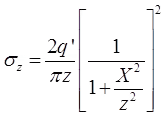where, σz = Vertical stress of any point having coordinate (x, z)
Load intensity = q' / m
at X = 0
σ= 2q' / πz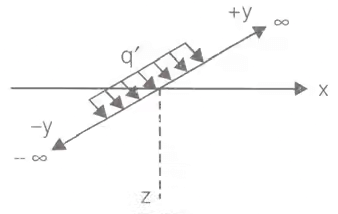(ii) Vertical Stress due to Strip Loading
σ= 2q' / π((X / B)(α) - (sin2β / 2))
where, σz = Vertical stress at point 'p'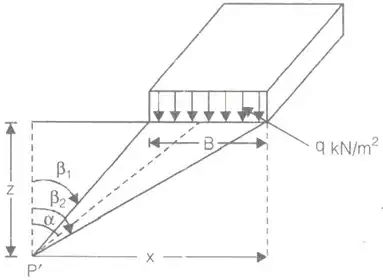(iii) σ= q / π[β + sinβ]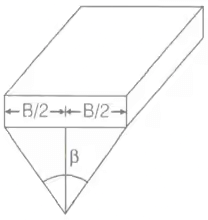(iv) Vertical stress below uniform load acting on a circular area.
σz = q(1 - cos3 θ)
where, cosθ =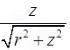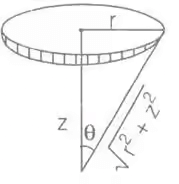Newmark's Chart Method (Uniform Load on irregular Areas)
• Newmark (1942) constructed influence chart , based on the Boussinesq solution to determine the vertical stress increase at any point below an area of any shape carrying uniform pressure.
• This method is applicable to semi-infinite, homogeneous, isotropic and elastic soil mass. It is not applicable for layered structure.
• The greatest advantage of this method is that it can be applied for a uniformly distributed area of an irregular shape.
• Chart consists of influence areas which have an influence value of 0.005 per unit pressure.
• Position the loaded area on the chart such that the point at which the vertical stress required is at the centre of the chart.
• Newmark's chart is made of concentric circles and radial lines. Normally there are 10 concentric circles and 20 radial lines.
• No. of concentric circle = 10
No. of radial lines = 20

Influence of area (1) = Influence of area (2) = Influence of area (3)
Influence of each area
= 1 / Total no. of sectoral area = 0.005
σz = 0.005qNA
where, NA = Total number of sectorial area of Newmark's chart.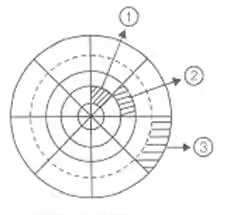Approximate method

σ= σz1 + σz2 + σz3 + ...
where,
σz1 = kB1(Q1/Z2)σz2 = kB2 . (Q22/Z2) ...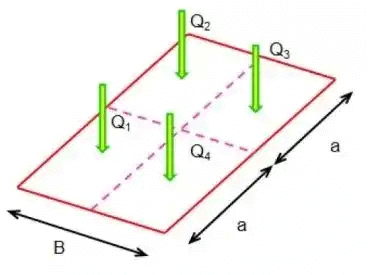2. Trapezoidal Method
σz at depth 'z' = q(BxL) / (B + 2ηz)(L + 2ηz)
For 1H : 1 V
σz = q(BxL) / (B + 2z)(L + 2z)
σz = q(BxL) / (B + 4z)(L + 4z)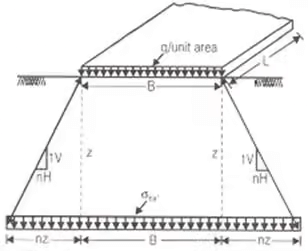3. Stress Isobar Method
Area bounded by 0.2q stress isobar is considered to be stressed by vertical stress on loading.
0.2q = 20% Stress Isobar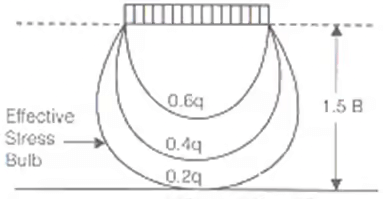Q. A concentrated load of 22.5 KN acts on thee surface of a homogeneous soil mass of large extent. Find the stress intensity at a depth of 15 metres and (i) directly under the load, and (ii) at a horizontal distance of 7.5 metres. Use Boussinesq's equations.
Ans:
According to Boussinesq's theory,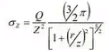r = 0; ∴r/z = 0
z = 15m, Q = 22.5 KN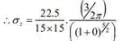= 47.75 N/m2
(ii) At a horizontal distance of 7.5 metres:
r = 7.5m, z = 15m
r/z = 7.5/15 = 0.5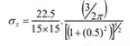= 27.33 N/m

The document Vertical Stress in Ground Notes | Study Soil Mechanics - Civil Engineering (CE) is a part of the Civil Engineering (CE) Course Soil Mechanics.
All you need of Civil Engineering (CE) at this link: Civil Engineering (CE)Use Code STAYHOME200 and get INR 200 additional OFF

## Soil Mechanics

31 videos|49 docs|42 tests

Track your progress, build streaks, highlight & save important lessons and more!

,

,

,

,

,

,

,

,

,

,

,

,

,

,

,

,

,

,

,

,

,

;